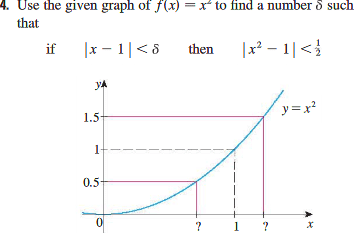# Prove that (|x-1| < delta) implies (|x^2 - 1| < 1/2)

## Homework Statement

"Use the given graph of f(x) = x^2 to find a number delta such that..."## Homework Equations

If ##([\lim_{x \rightarrow a} f(x)] - L)## then there exists an ##\epsilon## such that ##[0 < |x - a| < \delta] \Rightarrow [|f(x) - L| < \epsilon]##.
Here, f(x) and ##\epsilon## are given. So we have to find a ##\delta## such that ##|x-1|<\delta## implies ##|x^{2}-1| < \frac{1}{2}##.

## The Attempt at a Solution

I assume that we have to relate ##x^{2}-1## with ##\delta## somehow. I tried to do it like this:
Prove that ##(|x-1| < \delta) \Rightarrow (|x^{2}-1|<\frac{1}{2})##.
Choose ##\delta##:
##|x^{2}-1|<\frac{1}{2}##
##\Rightarrow -\frac{1}{2}<x^{2}-1<\frac{1}{2}##
##\Rightarrow -\frac{1}{2}+1<x^{2}<\frac{1}{2}+1##
##\Rightarrow \frac{1}{2}<x^{2}<\frac{3}{2}##
##\Rightarrow \sqrt{\frac{1}{2}}<x<\sqrt{\frac{3}{2}}##

But here I get stuck. I feel that I have to relate this to ##\delta##, but I don't know how!

As an aside, I can do regular ##\delta-\epsilon## proofs with linear equations now, so by rights this should be easy. But for some reason, it isn't.

vela
Staff Emeritus
Homework Helper
Try factoring ##x^2-1## and find an upper bound for x+1.

LCKurtz
Homework Helper
Gold Member
I don't think the question is asking you to work out a full ##\delta,~\epsilon## argument. Look at your picture and fill in the numbers for the ? marks. Then answer the question. It is there in the picture.

I don't think the question is asking you to work out a full ##\delta,~\epsilon## argument. Look at your picture and fill in the numbers for the ? marks. Then answer the question. It is there in the picture.

D'oh! I didn't even realize that it was that simple. I was overthinking it. Obviously, the two ##\delta## values are ##\sqrt{0.5}## and ##\sqrt{1.5}##, which ##\approx## 0.70 and 1.22 respectively. Plug either of these into the original function, subtract one, and take the absolute value, and you're left with .51 and 0.4484 So choose 1.22 for ##\delta## and you're good. I figured this out with a rather long ##\delta-\epsilon## argument below, in which I wandered about and "took the scenic route," as the saying goes (I'm not good at math yet, in case you can't tell), but I did figure it out eventually.

Try factoring ##x^2-1## and find an upper bound for x+1.
Okay, let's try that. We have ##|x^{2}-1|<\frac{1}{2}##. So let's factor: ##|(x+1)(x-1)|<\frac{1}{2}##. We can now write ##|x-1|<\frac{\frac{1}{2}}{x+1}##, and simplify that to ##|x-1|<\frac{1}{2x+2}##. We can now find an upper bound for ##|x+1|## with a quadratic equation. We know that ##|x-1| < \frac{1}{2x+2}##, so to find an upper bound we simply set ##|x-1| = \frac{1}{2x+2}##, and multiply both sides by ##2x+2## to obtain ##|x-1|(2x+2) = 1##. Subtract 1 and simplify the expression to obtain ##2x^{2}-2x+2x-2-1 = 0##. Combine like terms to get ##2x^{2}-3 = 0##. Now for the quadratic: ##(2x^{2}-3 = 0) \Rightarrow (x = \frac{0 \pm \sqrt{0^{2} - (4 \cdot 2 \cdot -3)}}{4}) ##. This simplifies to ##x = \frac{0 \pm \sqrt{24}}{4} = \frac{\pm 2\sqrt{6}}{4} = \frac{\pm \sqrt{6}}{2} ##. (notice that ##\frac{\sqrt{6}}{2}## is equal to ##sqrt{1.5}##. We now have an upper bound for x. We want a ##\delta > 0##, so drop the ##\pm## sign and just use choose any ##\delta < \frac{\sqrt{6}}{2}##.
To see this in action, let's choose ##x = \frac{\sqrt{6}}{2}## and use the limit definition, with ##<## changed to ##\leq## because we're finding an upper bound here: ##(|x^{2}-1| \leq \frac{1}{2}) \Rightarrow (|\frac{\sqrt{6}}{2}^{2} - 1| \leq \frac{1}{2}) \Rightarrow (\frac{6}{4}-1 \leq \frac{1}{2}) \Rightarrow (\frac{1}{2} \leq \frac{1}{2})##. So take ##\delta = 1.22## and you're good. Success!

LCKurtz